# 求二维函数Z=g(X,Y)型，用卷积公式求概率密度，积分区域如何确定（中）

### 求二维函数Z=g(X,Y)型，用卷积公式求概率密度，积分区域如何确定（中）

 ####  =======


#### 【例二】

(1)求P{X>2Y}
(2)求Z=X+Y的密度函数${f}_{z}\left(z\right)$$f_{z}(z)$

【解】

(1)求P{X>2Y}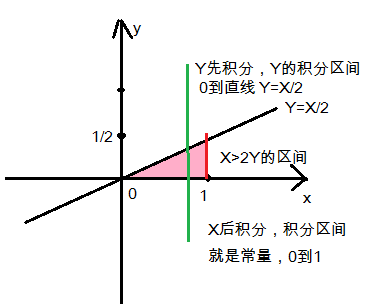$P\left(X>2Y\right)=\int {\int }_{X>2Y}f\left(x,y\right)dxdy$$P(X>2Y)=\int \int _{X>2Y} f(x,y)dxdy$
$={\int }_{0}^{1}dx{\int }_{0}^{\frac{x}{2}}f\left(x,y\right)dy$$=\int_{0}^{1}dx\int_{0}^{\frac{x}{2}}f(x,y)dy$
$={\int }_{0}^{1}dx{\int }_{0}^{\frac{x}{2}}\left(2-x-y\right)dy={\int }_{0}^{1}dx\left(\left(2-x\right)y-\frac{{y}^{2}}{2}\right){|}_{0}^{\frac{2}{x}}$$=\int_{0}^{1}dx\int_{0}^{\frac{x}{2}}(2-x-y)dy = \int_{0}^{1}dx ((2-x)y-\frac{y^2}{2})|^\frac{2}{x}_0$
$={\int }_{0}^{1}\left(x-\frac{5}{8}{x}^{2}\right)dx=\left(\frac{{x}^{2}}{2}-\frac{5}{8}\ast \frac{{x}^{3}}{3}\right){|}_{0}^{1}=\frac{1}{2}-\frac{5}{8}\ast \frac{1}{3}=\frac{7}{24}$$=\int_{0}^{1}(x-\frac{5}{8}x^2)dx = (\frac{x^2}{2}-\frac{5}{8}*\frac{x^3}{3})|^1_0=\frac{1}{2}-\frac{5}{8}*\frac{1}{3}=\frac{7}{24}$

(2)求Z=X+Y的密度函数${f}_{z}\left(z\right)$$f_{z}(z)$

Zmin=X+Ymin =X+0
Zmax=X+Ymax =X+1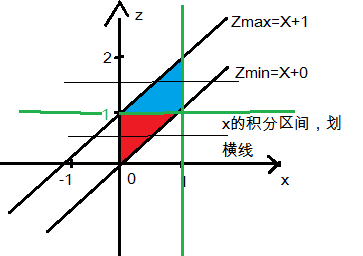${f}_{Z}\left(z\right)={\int }_{-\mathrm{\infty }}^{\mathrm{\infty }}f\left(x,z-x\right)dx$$f_{Z}(z) = \int_{-\infty}^{\infty}f(x,z-x)dx$

$0⩽z<1$$0\leqslant z<1$, 对x先积分，x的积分区间为红色区块，x的边界为0到Zmin直线，所以积分区间就是0到z
${f}_{Z}\left(z\right)={\int }_{0}^{z}\left(2-x-\left(z-x\right)\right)dx={\int }_{0}^{z}\left(2-z\right)dx=\left(2-z\right)x{|}_{0}^{z}=\left(2-z\right)\ast z$$f_{Z}(z)=\int_{0}^{z}(2-x-(z-x))dx=\int_{0}^{z}(2-z)dx=(2-z)x|^z_0=(2-z)*z$

$1⩽z<2$$1\leqslant z<2$, 对x先积分，x的积分区间为蓝色区块，x的边界为Zmax直线到1，所以积分区间就是z-1到1
${f}_{Z}\left(z\right)={\int }_{z-1}^{1}\left(2-x-\left(z-x\right)\right)dx={\int }_{z-1}^{1}\left(2-z\right)dx=\left(2-z\right)x{|}_{z-1}^{1}=\left(2-z{\right)}^{2}$$f_{Z}(z)=\int_{z-1}^{1}(2-x-(z-x))dx=\int_{z-1}^{1}(2-z)dx=(2-z)x|^1_{z-1}=(2-z)^2$

####  =====


#### 【例三】

【解】

[分析]

P{ X=k }=$\frac{\left(\lambda t{\right)}^{k}}{k!}\ast {e}^{-\lambda t}$$\frac{ ({\lambda t})^k} {k!}*e^{-\lambda t}$
“所谓指数分布就是求事件发生的时间间隔”
$P\left(X=0,N\left(t\right)\right)=\frac{\left(\lambda t{\right)}^{0}}{0!}\ast {e}^{-\lambda t}={e}^{-\lambda t}$$P(X=0,N(t)) =\frac{(\lambda t)^0}{0!}*e^{-\lambda t}=e^{-\lambda t}$
“在t时间内出现一个以上的概率”
$P\left\{X>0,N\left(t\right)\right\}=1-{e}^{-\lambda t}$$P\{ X>0,N(t) \}=1-e^{-\lambda t}$

$F\left(x<1000\right)=1-{e}^{-\lambda x}=1-\frac{1}{{e}^{\lambda x}}$$F(x<1000)=1-e^{-\lambda x} =1-\frac{1}{e^{\lambda x}}$
$=1-\frac{1}{{e}^{0.001\ast 1000}}=1-\frac{1}{e}$$= 1 - \frac{1}{e^{0.001*1000}} = 1-\frac{1}{e}$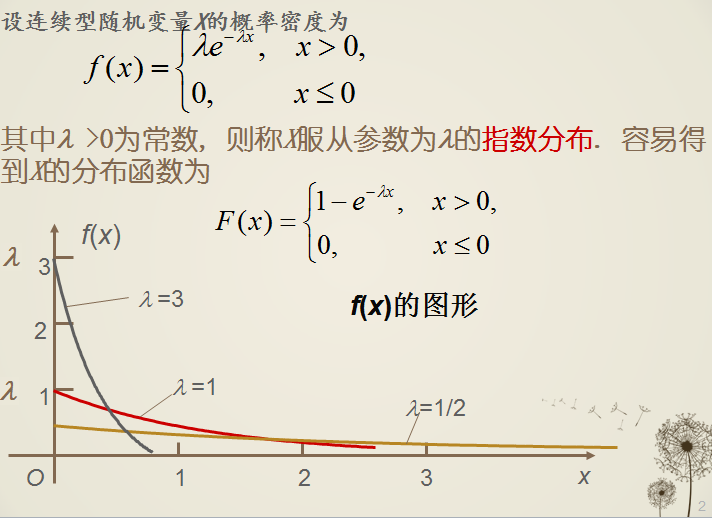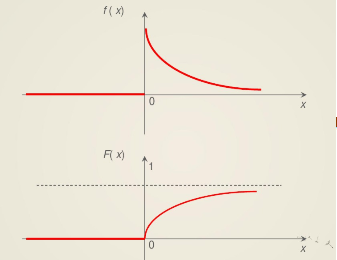=====

$其意义是指在x时间内事件发生的概率，其等于t时间内出现一个以上的概率，等于P\left\{X>0,N\left(t\right)\right\}=1-{e}^{-\lambda t}$${\color{Red} {其意义是指在 x时间内事件发生的概率}}，其等于t 时间内出现一个以上的概率，等于P\{ X>0,N(t) \}=1-e^{-\lambda t}$

${F}_{T}\left(t\right)=1-（1-{F}_{1}\left(t\right)）\ast （1-{F}_{2}\left(t\right)）\ast （1-{F}_{3}\left(t\right)）$$F_{T}(t) = 1-（1-F_{1}(t)）*（1-F_{2}(t)）*（1-F_{3}(t)）$
$=1-\left({e}^{-\lambda t}{\right)}^{3}$$=1 - ( e^{-\lambda t}) ^3$ (1)

${F}_{T1}\left(t\right)={F}_{1}\left(t\right)\ast {F}_{2}\left(t\right)\ast {F}_{3}\left(t\right)$$F_{T1}(t) = F_{1}(t)*F_{2}(t)*F_{3}(t)$
$=\left(1-{e}^{-\lambda t}{\right)}^{3}$$= ( 1-e^{-\lambda t}) ^3$
=$1-3{e}^{-\lambda t}+3{e}^{-2\lambda t}-{e}^{3\lambda t}$$1-3e^{-\lambda t}+3e^{-2\lambda t} -e^{3\lambda t}$ = $1-3{e}^{-\lambda t}\left(1-{e}^{-\lambda t}\right)-{e}^{3\lambda t}$$1-3e^{-\lambda t}(1-e^{-\lambda t})-e^{3\lambda t}$ (2)

${F}_{T1}\left(t\right)$$F_{T1}(t)$ 小于 ${F}_{T}\left(t\right)$$F_{T}(t)$ ， 而F(t)的含义是在t时间前系统发生故障的概率， 而实际上要求三个元件同时不发生故障系统才算不发生故障， 所以F(t)应该取大才对。

${F}_{M}\left(z\right)={F}_{X}\left(z\right)\ast {F}_{Y}\left(z\right),{F}_{N}\left(z\right)=1-\left[1-{F}_{X}\left(z\right)\right]\left[1-{F}_{Y}\left(z\right)\right]$$F_{M}(z)=F_{X}(z)*F_{Y}(z) , F_{N}(z)=1-[1-F_{X}(z)][1-F_{Y}(z)]$

#### 【例四】

【 解 】

X和Y的均匀分布的概率密度函数 = $\frac{1}{分布区域的面积}=\frac{1}{2}$$\frac{1}{分布区域的面积}=\frac{1}{2}$

$Z=X+Y型的密度函数为，{f}_{Z}\left(z\right)={\int }_{-\mathrm{\infty }}^{\mathrm{\infty }}f\left(x,z-x\right)dx$${\color{Red} {Z=X+Y型的密度函数为，f_{Z}(z)=\int_{-\infty}^{\infty}f(x,z-x)dx}}$

$Z=X/Y型的密度函数为，{f}_{Y/X}\left(z\right)={\int }_{-\mathrm{\infty }}^{\mathrm{\infty }}|x|f\left(x,zx\right)dx$${\color{Red} {Z=X/Y型的密度函数为，f_{Y/X}(z)=\int_{-\infty}^{\infty}|x|f(x,zx)dx}}$

$Z=XY型的密度函数为，{f}_{YX}\left(z\right)={\int }_{-\mathrm{\infty }}^{\mathrm{\infty }}\frac{1}{|x|}f\left(x,\frac{z}{x}\right)dx$${\color{Red} {Z=XY型的密度函数为，f_{YX}(z)=\int_{-\infty}^{\infty}\frac{1}{|x|}f(x,\frac{z}{x})dx}}$

$Z=XY型的密度函数为，{f}_{YX}\left(z\right)={\int }_{-\mathrm{\infty }}^{\mathrm{\infty }}\frac{1}{|x|}f\left(x,\frac{z}{x}\right)dx$$Z=XY型的密度函数为，f_{YX}(z)=\int_{-\infty}^{\infty}\frac{1}{|x|}f(x,\frac{z}{x})dx$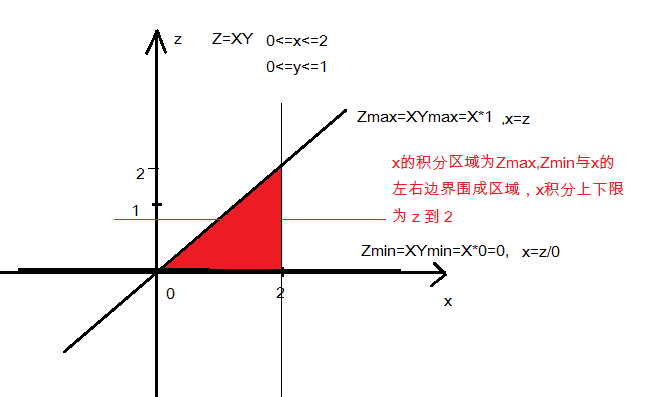Zmax = X*Ymax = X*1 =X, ==> 直线边界：x=z
Zmin = X*Ymin = X*0 =0 , ==> 直线边界：z=0
X的左右边界为 0 到 2

$0⩽z<2时，{f}_{XY}\left(z\right)={\int }_{-\mathrm{\infty }}^{\mathrm{\infty }}\frac{1}{|x|}f\left(x,\frac{z}{x}\right)dx={\int }_{z}^{2}\frac{1}{|x|}\ast \frac{1}{2}dx$$0\leqslant z<2 时， f_{XY}(z)=\int_{-\infty}^{\infty}\frac{1}{|x|}f(x,\frac{z}{x})dx = \int_{z}^{2}\frac{1}{|x|}*\frac{1}{2}dx$

${f}_{XY}\left(z\right)=\left\{\begin{array}{ccc}& \frac{1}{2}\left(ln2-lnz\right)& ,0⩽z<2\\ & 0& ,else\end{array}$

#### 参考书目：